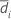Methods and formulas for Test mean - reference mean for Equivalence Test for a 2x2 Crossover Design

The following methods and formulas are used for testing the difference between the test mean and the reference mean.

Difference, standard error, and within-subjects standard deviation

Difference

The difference, D, for the test of equivalence is given by the following:

SE

The standard error for the difference is given by the following: where Sp is the pooled standard deviation, which is given by the following:

Within-subject standard deviation

The within-subject standard deviation, Sw, is given by the following:

Notation

TermDescriptionSample mean for sequence i (for more information, go to Methods and formulas for common concepts used in Equivalence Test for a 2x2 Crossover Design)
niNumber of participants in sequence i
SiSample standard deviation offor sequence i

Confidence interval

100(1-α)% CI

By default, Minitab uses the following formula to calculate the 100(1 – α)% confidence interval (CI) for equivalence:

CI = [min(C, Dl), max(C, Du)]

where:

100(1-2α)% CI

If you select the option to use the 100(1 – 2α)% CI, then the CI is given by the following formula:

CI = [Dl, Du]

One-sided intervals

For a hypotheses of Test mean > reference mean or Test mean - reference mean > lower limit, the 100(1 – α)% lower bound is equal to DL.

For a hypothesis of Test mean < reference mean or Test mean - reference mean < upper limit, the 100(1 – α)% upper bound is equal to DU.

Notation

TermDescription
DDifference between the test mean and the reference mean
SEStandard error
δ1Lower equivalence limit
δ2Upper equivalence limit
vDegrees of freedom
αThe significance level for the test (alpha)
t1-α, vUpper 1 – α critical value for a t-distribution with v degrees of freedom

T-values

Let t1 be the t-value for the hypothesis,, and let t2 be the t-value for the hypothesis,, whereis the difference between the mean of the test population and the mean of the reference population. By default, the t-values are calculated as follows:

For a hypothesis of Test mean > reference mean, δ1 = 0.

For a hypothesis of Test mean < reference mean, δ 2 = 0.

Notation

TermDescription
DDifference between the sample test mean and the sample reference mean
SEStandard error of the difference
δ1Lower equivalence limit
δ2Upper equivalence limit

P-values

The probability, PH0, for each null hypothesis (H0) is given by the following:
H0 P-ValueNotation

TermDescriptionUnknown difference between the mean of the test population and the mean of the reference population
δ1Lower equivalence limit
δ2Upper equivalence limit
vDegrees of freedom
Tt-distribution with v degrees of freedom
t1 t-value for the hypothesist2 t-value for the hypothesisNote

For information on how the t-values are calculated, see the section on t-values.

By using this site you agree to the use of cookies for analytics and personalized content.  Read our policy